### Murthy’s PPS Method

Murthy’s method (METHOD=PPS_MURTHY) selects two units from each stratum, with probability proportional to size and without replacement. The selection probability for unitin stratumequals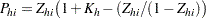where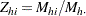and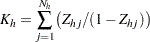Murthy’s algorithm first selects a unit with probability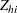. Then a second unit is selected from the remaining units with probability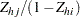, where unitis the first unit selected. The joint selection probability for unitsandin stratumequals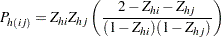See Cochran (1977, pp. 263–265) and Murthy (1957) for details.